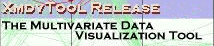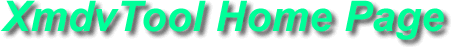Geochemical data: heavy metals in moss.

The dataset includes moss samples from 3 surveys (years 1985, 1990, 1995) over the area of 300 x 300 kilometers. Total number of points is 521, and the variables include X, Y (coordinates, m), Cu (copper), Ni (nickel), Pb (lead), V (vanadium), Zn (zinc)(contents, ppm=parts per million), year of sampling (1=1985, 2=1990, 3=1995). The purpose of sampling was to monitor the load of heavy metal pollution from atmosphere. The concentrations vary from 0 to maximum 114 ppm (Figure 1).

The easiest way to analyse time-related spatial data is to use statistics and GIS. Simple statistics, like histograms, can give an idea about the distribution of values, but cannot account for any spatial features. To produce a nice map representation with GIS needs the raw data to be interpolated into a grid. Time-series analysis can be applied only when the same locations are sampled each year, or when the data has been interpolated first. Considering the sparse and irregular sampling, and that different locations were sampled during three surveys, any kind of interpolation would introduce errors and uncertainties into the data layers. Further analysis with interpolated data would not give reliable results. This is especially true when the concentrations of metals are relatively low.

What one would like to get out from the analysis of the data is:

1) identify and deal with outliers (almost always present in geochemical data),

2) query high-valued samples and their location within the area,

3) visualize trends over the years.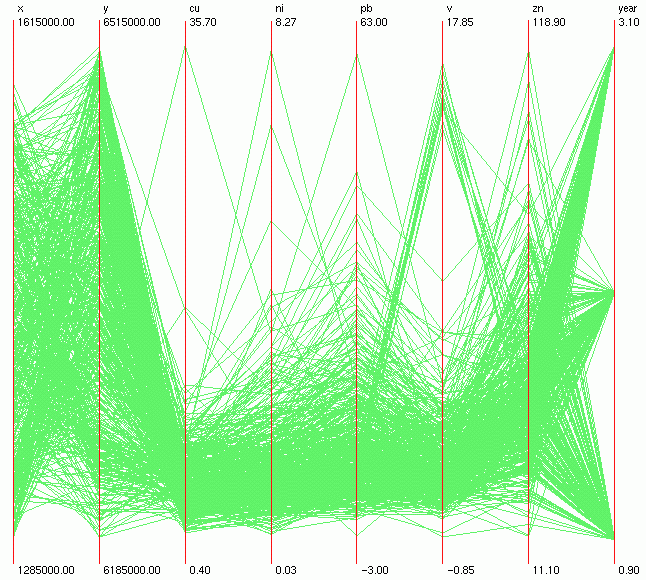Figure 1.

Figure 2.

Regarding outliers, the highest value for Cu (about 34 ppm) seems to compress the rest of the samples that are in the low range (Figure 1). However, brushing that maximum value can show that the same sample contains high amounts of other elements as well (and maximum for Pb). Instead of removing the multivariate outlier, we can leave this sample out and rescale the axes for Cu and Pb using their next highest values as maximum (Figure 3).

One cannot miss a nice cluster of samples with high levels of V (Figure 1, Figure 2). Brushing those samples (Figure 3, Figure 4) shows that they are all: related to year 1995, have the same composition concerning the levels of other metals, and are located in SW corner of the area. This could indicate a local source of point pollution that appeared only after 1990.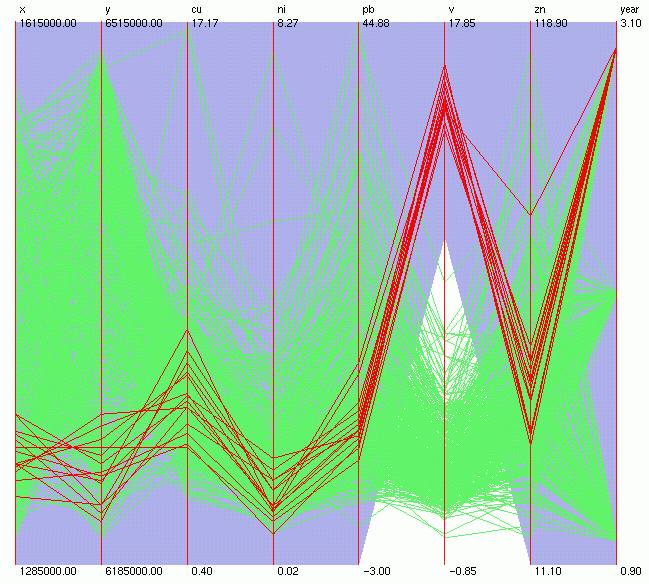Figure 3.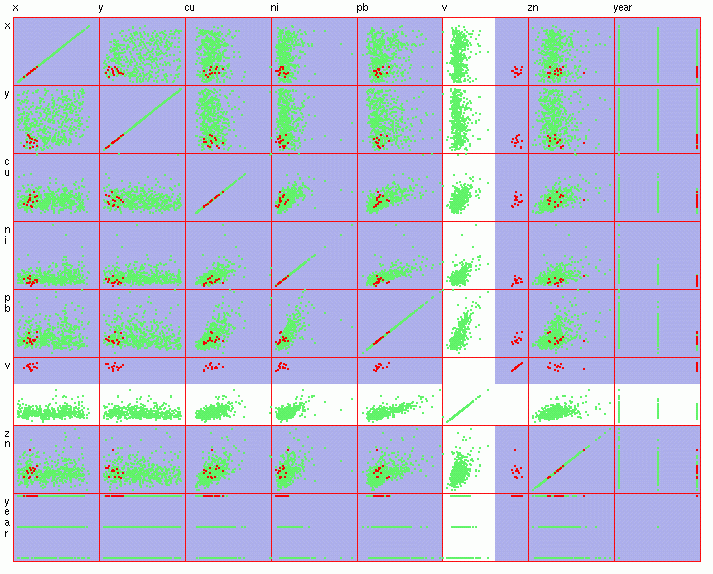Figure 4.

How are the levels of metals changing in time? After rescaling the V axis (and excluding the above-mentioned cluster) the samples can be brushed by year (Figure 5, Figure 6, Figure 7). We can see a decrease in the concentrations during the years, with levels of Pb and Ni showing the biggest drop.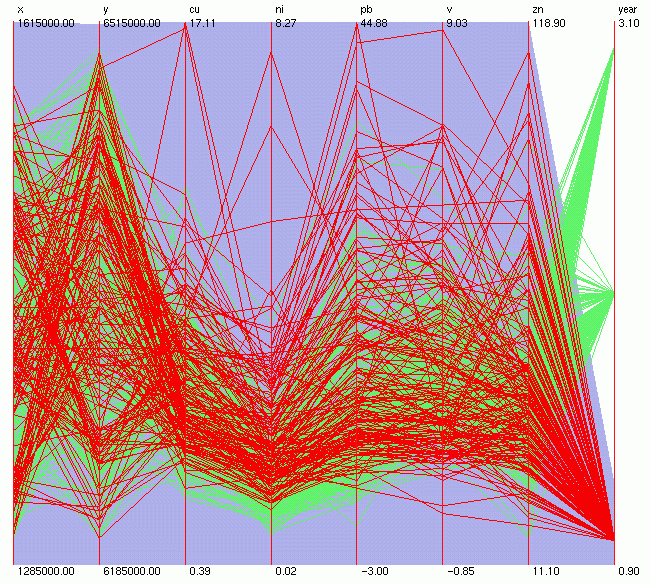Figure 5.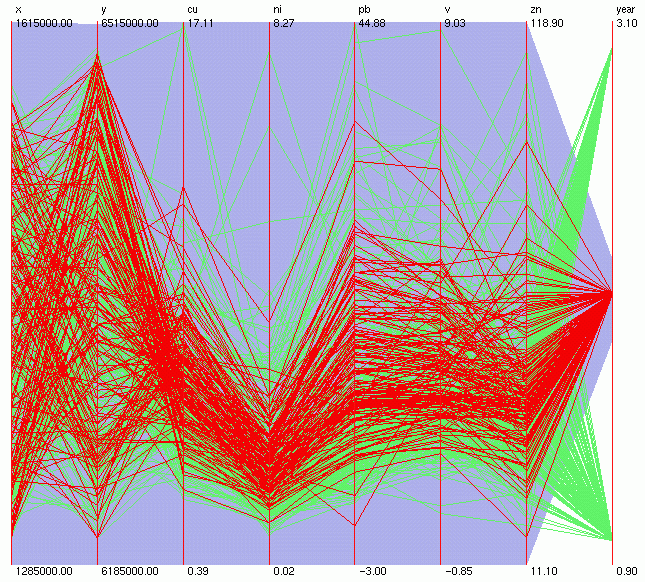Figure 6.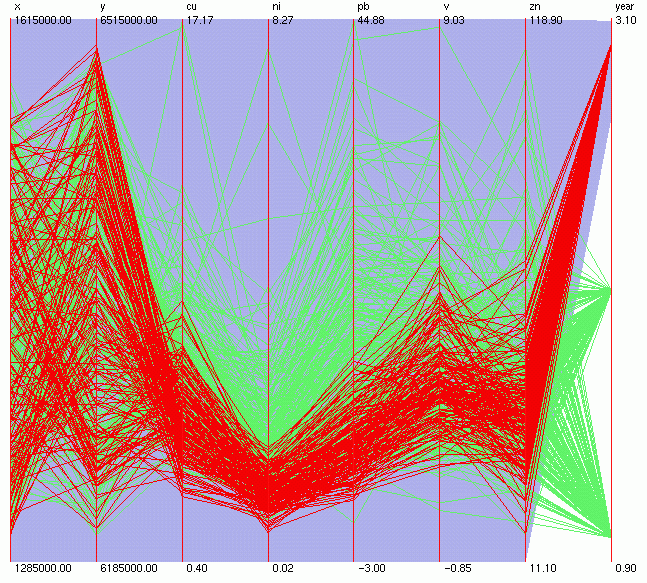Figure 7.

Data courtesy of SGU (the Geological Survey of Sweden).

Katrin Grunfeld

katring@geomatics.kth.s